# Semi-supervised pattern recognition in PPG signals

The purpose of this tutorial is to present self-training as a viable semi-supervised approach for regular pattern recognition in physiological signals, specifically using PPG or pulse oximetry, with only a few pre-labeled examples. The trained model can be applied during the signal pre-processing stage to distinguish relatively clean segments from ones affected by substantial noise and motion artifacts. It can achieve an observed accuracy as high as 94.7% using a limited initial training subset of 500 labels, and shows a performance increase compared to a 86-91% accuracy range achieved with fully supervised model trained against the entire available labeled subset.

## Code and usage

Main dependencies: Tensorflow/Theano, Keras, scikit-learn.

Clone the repo and call main.py with no arguments to train the model and visualize plots of the confusion matrix, loss over epochs, and a few example segments demonstrating the classifier results, as shown below. Note: the code was written and tested in Python 3.

## Data

The dataset contains ADC output values of PPG signals, recorded at 80Hz using the TI AFE4400 module, as well as timestamps and 3-axis accelerometer readings. Data was collected using wearable wristband sensors built and deployed as part of my research in the Social Computing group at the MIT Media Lab on data acquisition in early education environments, and were designed to track physiological processes in young children ages 3–8 (hence requiring miniature custom embedded devices). The dataset was recorded over a week at a Montessori preschool where children wore the bands while engaging in various types of activities, some inducing significant motion of the sensor unit that corrupted much of the data. This problem is very common in physiological signals recorded in unconstrained, real-world settings by wearable products, where free motion of the sensor unit is expected. PPG is especially prone to this, as it doesn’t benefit from the constant sensor-to-skin contact provided by gelled electrodes in common ECG/EKG monitors.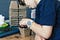The device worn by one of the children during school activity.

Increasing signal to noise ratio can be initially accomplished using a combination of a trans-impedance amplifier in the embedded system, followed by digital band-pass filters and a median filter for outlier removal. Subsequently, segmenting the data by discriminating parameters may be necessary to reject portions that include highly corrupted measurements, before relevant features can be extracted (e.g. heart rate variability, blood oxygen saturation).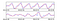Comparison of two types of labeled signals from the dataset, showing the original signal in blue, and a bandpass filtered version in red. Above: segment with high quality signal content — labeled positive. Below: segment content is random and should be rejected — labeled negative.

## Approach

We’ll use a binary classifier to distinguish between different types of signal segments, i.e. keepers vs. rejects (the latter being too corrupted), labeled as positive and negative respectively. Individually labeling segments by an analyst for a supervised algorithm can be tedious and time consuming as a large dataset is needed to represent diverse pattern components over the signal frequency spectrum. Instead, I applied a semi-supervised approach with a simple feedforward network to iteratively train the model in batches, firstly on the labeled subset, and then from the top few most confidently classified unlabeled examples. This allows the model to generalize better to the many small variations in the input data and prevent overfitting the initial labeled subset. A two layer neural network was used in order to capture subtler characteristics, and the use of gradient descent as a loss optimization function allows us to train in multiple iterations instead of having to feed the entire labeled training data at once. The benefits of the network over simpler models are discussed next.

As input vectors to the network, we’ll use the logarithm of the power spectral density (PSD) estimates for each 3.2 second/256 sample window. As we’re dealing with a non-stationary process, we’ll assume that the PPG is theoretically stationary during each segment window. This allows us to work in the frequency domain, which is generally simpler where possible.

The PSD is a useful feature tool when we care primarily about the general shape of the waveform but not as much about high frequency components. This is helpful for two reasons: firstly, the model is initially given a limited set of labeled examples and must generalize well to most possible variations in the test set. Secondly, PPG waveforms can exhibit a wide variety of differences in the high frequency components, depending on many individual and physical factors such as skin complexion, ambient light interference, recording area on the body, physical distance between the sensor and the skin, as well as the type of sensor (e.g. reflective vs. transmission).

To account for variation within the low frequency power components of the signal, normally occurring between 1 and 2Hz in people, we use the mean of the log PSDs in that range instead of the individual power in each frequency. This increases the similarity between clean signals with different heart rate frequencies.

Finally to further help with generalization, we’ll use principal components analysis (PCA) to constrain the PSD dimensionality to the 10 most important frequency components.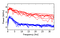The log PSD esimates of a few strongly separable clean signal segments (blue) and noisy (red). Apart from large variation across the frequency spectrum, the power in the high frequency range is greater in noisy the signals, as expected.

## Alternatives

Alternatives to classification: dynamic time warping is a useful time series method for measuring the similarity between signals that are out of phase and of different lengths, so long as one signal is roughly a time-stretched series of the other. As such, it’s a good option for detecting temporal feature similarity when given signals with an equal number of “beats” or peaks. Therefore, it isn’t suitable for classification of constant-length, variable-frequency segment classification. Instead, it’s possible to read the signal in using a window of N samples, where N is the length of the desired pattern at the lowest expected frequency, and perform DTW against a set of beat patterns of length N. This can be potentially effective and fast to compute, but depending on how varied the pattern shape is, might require a large number of comparisons. One application is anomaly detection in ECG, where the signal pattern largely conforms to a very specific shape defined by high frequency components. Hence accurate time series comparison in PPG-generated signals can be much more difficult to obtain than in ECG.

Standard supervised models: when using a fully supervised method using the same network (i.e. training with the pre-labeled examples only) an accuracy of roughly 86% was observed, which corresponds to the accuracy on the first iteration of the self training algorithm. The accuracy curve shown below demonstrates the increase in accuracy over each iteration. Other models such as support vector machines (SVM) or k-nearest neighbors (KNN) achieved a slightly improved accuracy of 88–89% using the initial labeled set. This demonstrates that with relatively few training examples (e.g. 1000) the network cannot learn as well as simpler models. However, when more labeled data is used (as generated in each iteration of the network) the network shows a clear advantage over other approaches.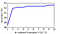Accuracy of the model against the test set per iteration. Self training can greatly improve the accuracy of the model in this case.

## Data Preprocessing

The data directory contains files associated with a particular device ID and a date on which the device was used, as indicated in each line of the datafiles. For consistency, all readings in the same file were from the same person on a single day, but files may be of different lengths. File 20 is reserved as the test set (can be set in classes/DataSource.py). positive_ranges.csv and negative_ranges.csv contain lines of file IDs, start, and end indices of segments previously labeled as either usable (positive) or corrupted (negative). These will be used when reading the signal data for the first training batch.

The gist of work done by load_or_process_labeled_dataset and load_or_process_entire_dataset is very similar. load_or_process_labeled_dataset reads in a number of labeled ranges from each class, while load_or_process_entire_dataset reads in all the remaining unlabeled data as signal segments directly and applies a preliminary median and lowpass filters. They then extract the PSD features from the corresponding signal segments, reduce the feature vector dimensionality using PCA, and standardize them to a zero mean and a unit standard deviation.

## Model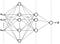Network architecture: an input layer followed by two hidden ones, and an output layer with a single output node.

Since we’re interested in training a classifier sequentially in batches (i.e. using stochastic gradient descent-type optimizer), we use a simple feedforward network consisting of 2 fully connected hidden layers, with 128 and 32 neurons respectively. We apply a ReLU activation function after each hidden layer, and a sigmoid function on the output layer to obtain a binary probability distribution. A decay rate of 0.05 is set on the optimizer in order to gradually reduce the effects of the parameter updates by training on data batches drawn later in the process. The logic here is that we trust our initial labeled dataset, but our confidence in the classifier’s prediction-generated labels decreases over each subsequent iteration. Also, this can effectively reduce the likelihood of the algorithm picking up inconsequential trends in the data from one batch and unintentionally carrying them into subsequent batches, and skewing the model parameters.

Keras is used to define the model in SignalClassifier, and we initiate it in main.py as shown below (hyperparameters chosen based on performance on a validation set):

## Algorithm

We start by training the model on the labeled subset, and for the 10 subsequent iterations, generate and sort by the probability predictions on the entire remaining data. We then draw the 500 most confidently classified feature vectors from each class, based on the prediction confidence rates, and refit the model. This way, the algorithm starts with only 1000 labeled examples, but generates labels for unlabeled examples in the dataset on every iteration, which it subsequently feeds as new training examples to the network. After 10 iterations, we’ve trained the algorithm on 10,000 examples in total.

To evaluate the model once done, we read in our test set file and call the SignalClassifier evaluate method, which classifies the examples based on an optimal threshold (determined using the Keras evaluate method).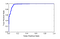ROC curve of test set classification results. Our test set contains roughly equal number of examples from each class, so a 0.5 true positive rate is an adequate value.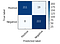Confusion matrix showing the classification performance on the test set.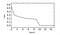Loss rate between epochs.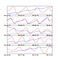Most confidently classified segments from the test set in their corresponding time ranges (HH:MM:SS). Original signals are blue and bandpass filtered are red.

Once trained, we can use the model to classify live streamed segments and apply peak analysis to calculate the heart rate BPM value. From there, plotting the heart rate variability is straightforward.

## Final Notes

• The accuracy (as measured by precision and recall) on any realistic test set naturally depends on the diversity and quality of the examples in the labeled subset that we initially train on. The script draws samples for this subset randomly, but the combination of samples can greatly affect results, especially when only a few of them are used (under 100 could be considered danger zone, but try and let me know if you achieve adequate results with even fewer initial samples). As a good rule of thumb, if the labeled subset size you define is small, ensure the subset on a whole encapsulates as many of the expected variations in your test set as possible.
• If any of the parameters to the DataSource object are changed, you’ll need to regenerate the pre-processed feature data files, stored in the project ./cache folder (calculating the PSD features for each segment in the entire dataset is slow, and only needs to be performed once after the parameters are changed). To regenerate the training set, pass the ‘regenerate’ flag when invoking the main.py script, which will take a few seconds to minutes in order to compute the feature vectors.
• To label new examples from the unlabeled dataset, I have provided label_data.py. Call the script with the -h option for usage instructions.